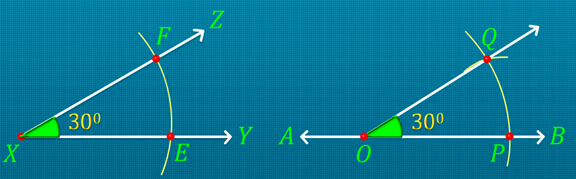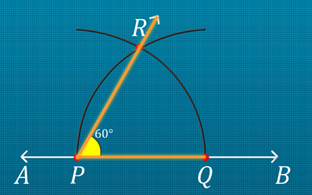Notes On Construction of Angles - CBSE Class 6 Maths
 Construction of copy of an angle: An exact copy of a line segment can be constructed using a ruler and a compass. Construction of a copy of an angle YXZ. Draw a line AB. Mark any point O on AB. Place the compass pointer at vertex X of the given figure and draw an arc with a convenient radius, cutting rays XY and XZ at points E and F, respectively. Without changing the compass settings, draw an arc on line AB from point O. It cuts line AB at P. Set the compass to length EF. Without changing the compass settings, draw an arc from P cutting the previous arc at point Q. Join points O and Q. Hence, ∠POQ  is the required copy of ∠YXZ.Construction of the bisector of an angle: Construction of the bisector of an angle LMN. Place the compass pointer at vertex M of the given angle. Draw an arc cutting rays ML and MN at U and V respectively. Draw an arc with V as the centre and a radius more than half the length of UV in the interior of ∠LMN. Draw another arc with U as the centre and the same radius intersecting the previous arc. Name the point of intersection of the arcs as X. Join points M and X. Ray MX is the required bisector of ∠LMN. Construction of a 60° angle: Draw a line. Mark a point P on the line. Draw an arc from point P with a convenient radius cutting the line at a point. Name the point of intersection of the arc and the line as Q. Draw another arc with Q as the centre and the same radius so that it passes through point P. Name the point of intersection of the two arcs as R. Join points P and R. Ray PR forms an angle with ray PQ at point P, which measures 60°. ∠QPR is the required angle measuring 60°.Construction of a 30° angle: To obtain a 30° angle, we need to bisect a 60° angle. Draw an arc with Q as the centre and a radius more than half the length of QR. Draw another arc with R as the centre without changing the compass settings so that it intersects the previous arc. Name the point of intersection of the arcs as S. Join points P and S. ∠QPS is the required angle measuring 30°. In a similar way, we can construct a 120° angle and 90° angle without using the protractor. Construction of a 120° angle Draw line XY. Mark a point on the line and name it as P. Draw an arc with P as the centre and a convenient radius so that it cuts the line at Q. Draw another arc with Q as the centre without changing the compass settings so that it intersects the first arc at R. Draw another arc with R as the centre without changing the compass settings so that it intersects the first drawn arc at point S. Join points P and S. ∠SPQ is the required angle measuring 120°. Construction of a 90° angle Draw line l and mark point P on it. Draw an arc with P as the centre and a convenient radius cutting line l at Q. Draw another arc with Q as the centre and the same radius cutting the first arc at R. Draw an arc with R as the centre and the same radius cutting the first arc at S. Join points P and R. Join points P and S. 90° lies to the centre of 60° and 120°. Draw an arc with R as the centre and a radius more than half the length of RS in the interior of ∠RPS. Draw another arc with S as the centre and the same radius so that it intersects the previous arc at T. Join points P and T. PT is the perpendicular line to PQ. ∠QPT is the required angle measuring 90°.

Summary

 Construction of copy of an angle: An exact copy of a line segment can be constructed using a ruler and a compass. Construction of a copy of an angle YXZ. Draw a line AB. Mark any point O on AB. Place the compass pointer at vertex X of the given figure and draw an arc with a convenient radius, cutting rays XY and XZ at points E and F, respectively. Without changing the compass settings, draw an arc on line AB from point O. It cuts line AB at P. Set the compass to length EF. Without changing the compass settings, draw an arc from P cutting the previous arc at point Q. Join points O and Q. Hence, ∠POQ  is the required copy of ∠YXZ.Construction of the bisector of an angle: Construction of the bisector of an angle LMN. Place the compass pointer at vertex M of the given angle. Draw an arc cutting rays ML and MN at U and V respectively. Draw an arc with V as the centre and a radius more than half the length of UV in the interior of ∠LMN. Draw another arc with U as the centre and the same radius intersecting the previous arc. Name the point of intersection of the arcs as X. Join points M and X. Ray MX is the required bisector of ∠LMN. Construction of a 60° angle: Draw a line. Mark a point P on the line. Draw an arc from point P with a convenient radius cutting the line at a point. Name the point of intersection of the arc and the line as Q. Draw another arc with Q as the centre and the same radius so that it passes through point P. Name the point of intersection of the two arcs as R. Join points P and R. Ray PR forms an angle with ray PQ at point P, which measures 60°. ∠QPR is the required angle measuring 60°.Construction of a 30° angle: To obtain a 30° angle, we need to bisect a 60° angle. Draw an arc with Q as the centre and a radius more than half the length of QR. Draw another arc with R as the centre without changing the compass settings so that it intersects the previous arc. Name the point of intersection of the arcs as S. Join points P and S. ∠QPS is the required angle measuring 30°. In a similar way, we can construct a 120° angle and 90° angle without using the protractor. Construction of a 120° angle Draw line XY. Mark a point on the line and name it as P. Draw an arc with P as the centre and a convenient radius so that it cuts the line at Q. Draw another arc with Q as the centre without changing the compass settings so that it intersects the first arc at R. Draw another arc with R as the centre without changing the compass settings so that it intersects the first drawn arc at point S. Join points P and S. ∠SPQ is the required angle measuring 120°. Construction of a 90° angle Draw line l and mark point P on it. Draw an arc with P as the centre and a convenient radius cutting line l at Q. Draw another arc with Q as the centre and the same radius cutting the first arc at R. Draw an arc with R as the centre and the same radius cutting the first arc at S. Join points P and R. Join points P and S. 90° lies to the centre of 60° and 120°. Draw an arc with R as the centre and a radius more than half the length of RS in the interior of ∠RPS. Draw another arc with S as the centre and the same radius so that it intersects the previous arc at T. Join points P and T. PT is the perpendicular line to PQ. ∠QPT is the required angle measuring 90°.

Previous
Next